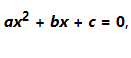Python Program to Solve Quadratic equation

In this program, you will learn how to calculate the roots of a square equation when the coefficients a, b and c are known.

The quadratic formula uses "a", "b" and "c" fromwhere "a", "b" and "c" are just numbers; They are the "numerical modules" of the square equation they have given you to solve.

To understand the following program, you must have the knowledge to follow Python concepts:

Introduction to Python

Basics of Python

For quadratic formula: forthe values of x which are the solutions of the equation are given as:Now take a look at how to write a program to solve Quadratic equation in Python Programming.

Python Code:

`import cmatha = float(input('enter value of a:'))b = float(input('enter value of b:'))c = float(input('enter value of c:'))def h(x):    a*x**2+b*x+c    return h(x)disc = b**2-4*a*croot1 = (-b+cmath.sqrt(disc))/2*aroot2 = (-b+cmath.sqrt(disc))/2*aprint(root1,root2)`
`Output:`
```enter value of a: 49
enter value of b: 9
enter value of c: 36
(-220.5+2046.153403340033j) (-220.5+2046.153403340033j)```
`Recommended Post:Program to Add Two Numbers in PythonProgram to check whether the number is prime Plotting of even, odd, prime numbers Subplot of even, odd and prime numbers from a list Python Program to print Arithmetic Progress seriesProgram to find even and odd numbers from the listProgram to check whether a number is odd or even in PythonPython Program to print and plot the Fibonacci SeriesProgram to find sum of the elements in PythonPython Program to print Geometric Progress seriesPython Program to Print Harmonic Progress seriesProgram to Plot Arithmetic Progression Series in PythonProgram to Plot Geometric Progression Series in PythonProgram to Plot Harmonic Progression Series in PythonSubplot of G.P, A.P, H.P and Fibonacci series in Python`